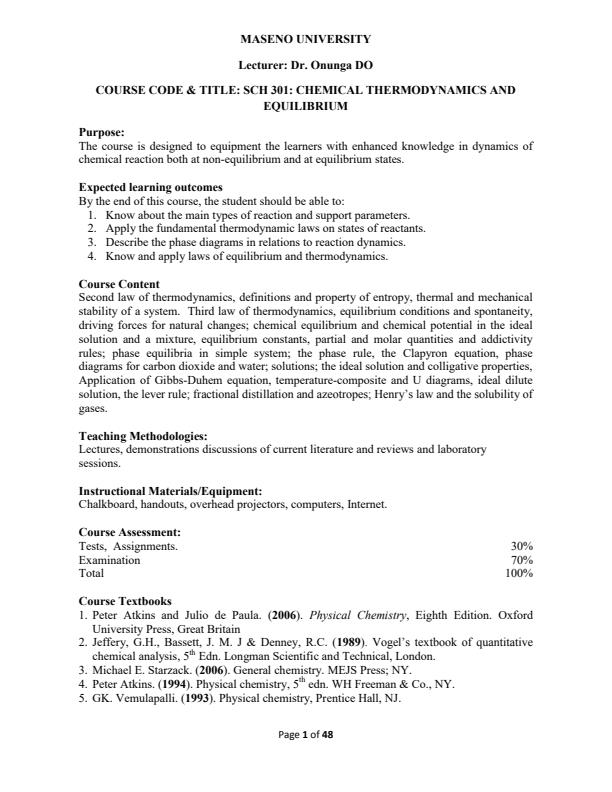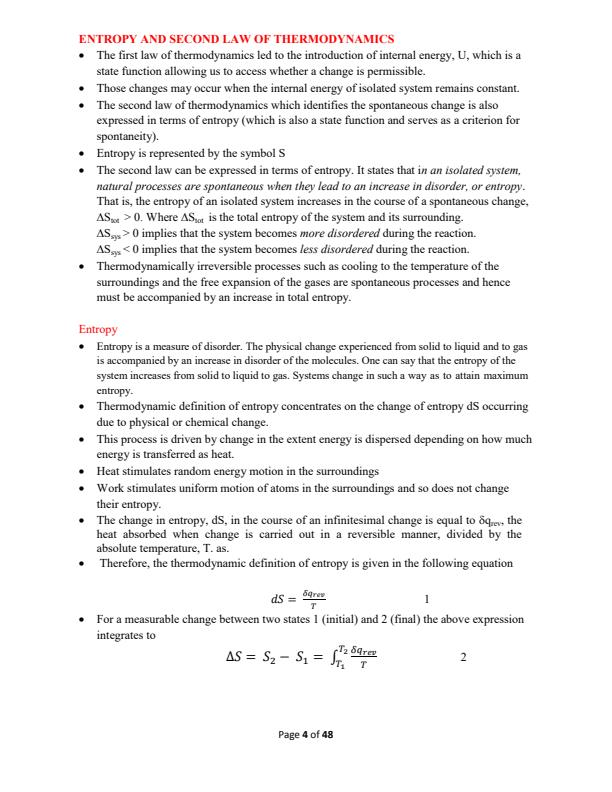# SCH 301: Chemical Thermodynamics and Equilibrium Notes

Institution: Maseno University

Course: Bachelor of Science

Posted By: 0724622244

Document Type: PDF

Number of Pages: 48

Price: KES 100

## Summary

Purpose:
The course is designed to equipment the learners with enhanced knowledge in dynamics of
chemical reaction both at non-equilibrium and at equilibrium states.
Expected learning outcomes
By the end of this course, the student should be able to:
1. Know about the main types of reaction and support parameters.
2. Apply the fundamental thermodynamic laws on states of reactants.
3. Describe the phase diagrams in relations to reaction dynamics.
4. Know and apply laws of equilibrium and thermodynamics.
Course Content
Second law of thermodynamics, definitions and property of entropy, thermal and mechanical
stability of a system. Third law of thermodynamics, equilibrium conditions and spontaneity,
driving forces for natural changes; chemical equilibrium and chemical potential in the ideal
solution and a mixture, equilibrium constants, partial and molar quantities and addictivity
rules; phase equilibria in simple system; the phase rule, the Clapyron equation, phase
diagrams for carbon dioxide and water; solutions; the ideal solution and colligative properties,
Application of Gibbs-Duhem equation, temperature-composite and U diagrams, ideal dilute
solution, the lever rule; fractional distillation and azeotropes; Henry?s law and the solubility of
gases.

Below is the document preview. Purchase to access the complete document.

••••........